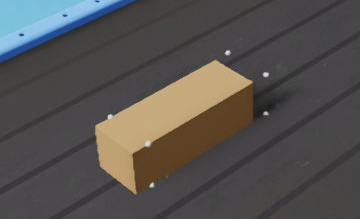# Computing vertices of a cuboid

I need to get the vertices of a cuboid on a conveyor belt and, since I couldn’t find an off-the-shelve function for this, I wrote my own. The problem I’m seeing with it, however, is that the vertices I get are delayed by one or more physics steps.

My approach to getting the vertices is to read the cuboid’s pose and its size (i.e. translation, rotation, and scale) at each physics step using the below functions.

``````# Get the orientation
o = prim.GetAttribute("xformOp:orient").Get()
real = o.GetReal()
imaginary = o.GetImaginary()
# convert to numpy array in the order [w, x, y, z]
rotation_wxyz = np.array([real, imaginary, imaginary, imaginary])

# Get the translation
t = prim.GetAttribute("xformOp:translate").Get()
# convert to numpy array
translation_xyz = np.array([t, t, t])

# Get the scale
s = prim.GetAttribute("xformOp:scale").Get()
# convert to numpy array
scale_xyz = np.array([s, s, s])
``````

This is sufficient information to compute the vertices with some trigonometry.

``````from scipy.spatial.transform import Rotation

# Vertices of the cuboid in the local frame
original_vertices = np.array([
[-scale_xyz / 2, -scale_xyz / 2, -scale_xyz / 2],
[scale_xyz / 2, -scale_xyz / 2, -scale_xyz / 2],
[scale_xyz / 2, scale_xyz / 2, -scale_xyz / 2],
[-scale_xyz / 2, scale_xyz / 2, -scale_xyz / 2],
[-scale_xyz / 2, -scale_xyz / 2, scale_xyz / 2],
[scale_xyz / 2, -scale_xyz / 2, scale_xyz / 2],
[scale_xyz / 2, scale_xyz / 2, scale_xyz / 2],
[-scale_xyz / 2, scale_xyz / 2, scale_xyz / 2]

])

# Convert the orientation to a rotation matrix with SciPy
r = Rotation.from_quat([rotation_wxyz, rotation_wxyz, rotation_wxyz, rotation_wxyz])

# Rotate the original vertices in the local frame
rotated_vertices = r.apply(original_vertices)

# Translate the rotated vertices
translated_rotated_vertices = rotated_vertices + translation_xyz
``````

However, when I visualize the vertices by translating spheres to the computed vertex locations (of the moving cuboid), I see that they lag behind wrt the actual vertices.

Is a bug? It seems that when requesting the translation, rotation, and scale of the cuboid, it communicates the values of the previous physics step.

Below is an example of this where the spheres represent the computed vertices of the cuboid.There are multiple reasons for this - We’d need to know what update event are you using to trigger your code execution - The simulation has it’s own event pipeline, so does rendering. If you are doing this on rendering pipeline, you’ll be one or more simulation steps behind indeed.

If you are on the simulation pipeline, you will still likely be one step behind, since rendering happens before user code in general, so your updated coordinates will only be added to the next frame. You can set your code priority to -1000 or something so it’s one of the first things that run on a simulation step, before it triggers rendering.

see Event streams — kit-manual 105.0.2 documentation for how to set the priority for your event trigger.